# Clustering around the rainbow ray

We want to show that for any point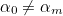the interval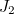coming from any small interval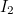centred on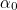is larger than the interval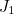coming from the interval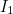of the same width as, but centred on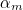(see the figure).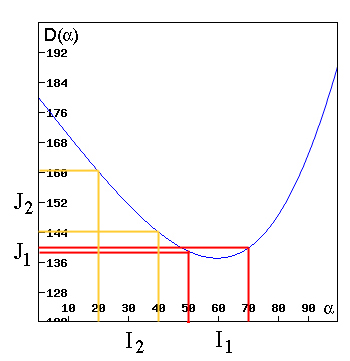Figure 7: The interval J1 is smaller than the interval J2.

Sinceis the only point with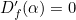we know that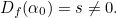To match with our figure we’ll assume that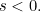An analogous argument works when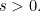Now choose a positive numberwith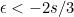(you’ll see later why we make this choice). From the definition of a derivative we know that there exists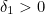such that for all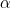with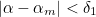we have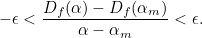(1)

Similarly, there exists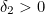such that for allwith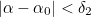we have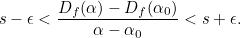(2)

Now choose any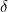less than both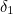and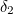. Letbe the interval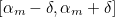and letbe the interval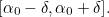Sinceis the only value for whichwe know that the maximum of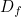onmust occur at one of the end-points of. Call that point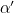. This means that the width of the intervalis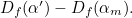Sinceis the only value for whichwe also know that the slope ofis negative throughout the interval. Onthe functionachieves its maximum at the lower end point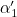ofand its minimum at the upper end point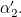The width of the intervalis therefore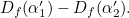Now since bothand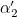lie withinof, we know from inequality 2 that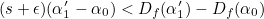and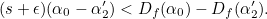(The inequalities have changed direction because, since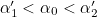, we have multiplied by a negative number in each case.)

Putting this together gives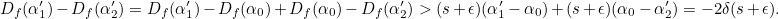(3)

Sincelies withinof, we know from inequality 1 that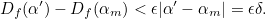(4)

And since we have chosenso that, putting inequalities 3 and 4 together gives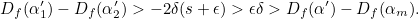Thus, intervalis wider than interval. QED.

Back to main article

• Want facts and want them fast? Our Maths in a minute series explores key mathematical concepts in just a few words.